# LeetCode通关：栈和队列六连，匹配问题有绝招

2021/8/20 15:22:24 浏览：

# 栈和队列基础

## 栈的结构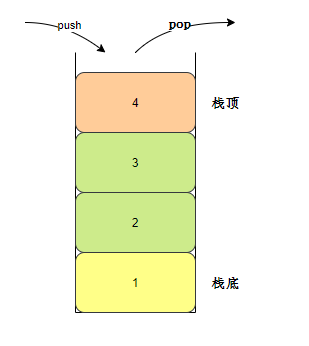## 栈的实现

``````Deque<TreeNode> stack = new LinkedList<TreeNode>();//类型为TreeNode
Stack<TreeNode> stack = new Stack<TreeNode>();
``````

## 队列结构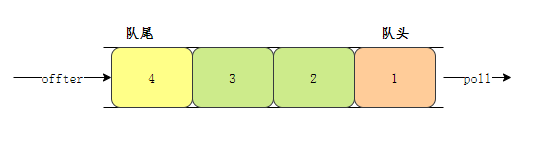## 队列实现

``````  Queue<TreeNode> queue = new LinkedList<TreeNode>();
``````

# 刷题现场

## 剑指 Offer 09. 用两个栈实现队列

☕ 题目：剑指 Offer 09. 用两个栈实现队列(https://www.cnblogs.com/lyck/p/14843843.html)

❓ 难度：简单

📕 描述：

``````输入：
[[],,[],[]]

``````

``````输入：
[[],[],,,[],[]]

``````

💡思路：

• tailStack 作为队尾，模拟入队操作，当有一个元素入队时，则将其 push 到tailStack 栈顶。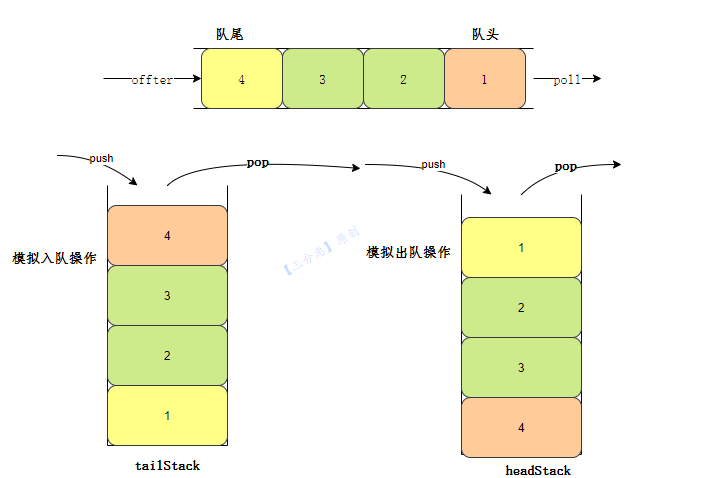``````public class CQueue {

//定义两个栈

public CQueue() {
}

//入队
public void appendTail(int value) {
//入队，往tailStack中压入值
tailStack.push(value);
}

//出队
//如果队头为空
while (!tailStack.isEmpty()) {
}
}
return -1;
}
}
}
``````

🚗 时间复杂度：入队O(1，出队O(n)。

🏠 空间复杂度：引入了两个栈，所以空间复杂度O(n)。

## LeetCode225. 用队列实现栈

☕ 题目：225. 用队列实现栈 (https://leetcode-cn.com/problems/implement-stack-using-queues/)

❓ 难度：简单

📕 描述：

• void push(int x) 将元素 x 压入栈顶。
• int pop() 移除并返回栈顶元素。
• int top() 返回栈顶元素。
• boolean empty() 如果栈是空的，返回 true ；否则，返回 false 。

• 你只能使用队列的基本操作 —— 也就是 `push to back``peek/pop from front``size` 和 `is empty` 这些操作。
• 你所使用的语言也许不支持队列。 你可以使用 list （列表）或者 deque（双端队列）来模拟一个队列 , 只要是标准的队列操作即可。

``````输入：
["MyStack", "push", "push", "top", "pop", "empty"]
[[], , , [], [], []]

[null, null, null, 2, 2, false]

MyStack myStack = new MyStack();
myStack.push(1);
myStack.push(2);
myStack.top(); // 返回 2
myStack.pop(); // 返回 2
myStack.empty(); // 返回 False
``````

💡 思路：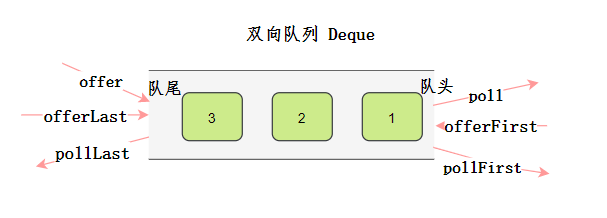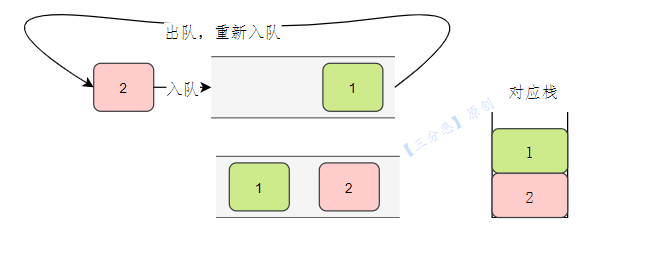``````public class MyStack {
//单向队列
Queue<Integer> queue;

/**
* Initialize your data structure here.
*/
public MyStack() {
}

/**
* Push element x onto stack.
*/
public void push(int x) {
//入队元素
queue.offer(x);
//将之前的元素，出队，重新入队
for (int i = 0; i < queue.size() - 1; i++) {
queue.offer(queue.poll());
}
}

/**
* Removes the element on top of the stack and returns that element.
*/
public int pop() {
return queue.poll();
}

/**
* Get the top element.
*/
public int top() {
return queue.peek();
}

/**
* Returns whether the stack is empty.
*/
public boolean empty() {
return queue.isEmpty();
}
}
``````

🚗 时间复杂度：入栈O(n），出栈O(1)。

🏠 空间复杂度：引入了队列，空间复杂度O(n)。

## LeetCode20. 有效的括号

☕ 题目：20. 有效的括号 (https://leetcode-cn.com/problems/valid-parentheses/)

❓ 难度：简单

📕 描述：

• 左括号必须用相同类型的右括号闭合。
• 左括号必须以正确的顺序闭合。

``````输入：s = "()"

``````

``````输入：s = "()[]{}"

``````

``````输入：s = "(]"

``````

``````输入：s = "([)]"

``````

``````输入：s = "{[]}"

``````

• `1 <= s.length <= 104`
• `s` 仅由括号 `'()[]{}'` 组成

💡 思路：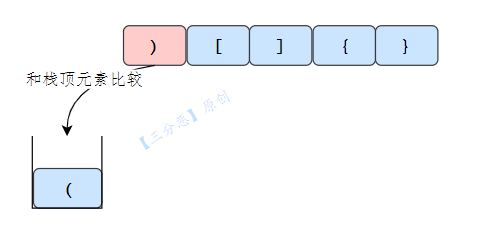``````    public boolean isValid(String s) {
//遍历字符串
for (int i = 0; i < s.length(); i++) {
char c = s.charAt(i);
//遇到左括号，入栈
if (c == '(' || c == '[' || c == '{') {
stack.push(c);
}
//右括号匹配
if (c == ')') {
if (stack.isEmpty() || stack.pop() != '(') {
return false;
}
}
if (c == ']') {
if (stack.isEmpty() || stack.pop() != '[') {
return false;
}
}
if (c == '}') {
if (stack.isEmpty() || stack.pop() != '{') {
return false;
}
}
}
return stack.isEmpty();
}
``````

🚗 时间复杂度：O(n）。

🏠 空间复杂度：O(n)。

## LeetCode1047. 删除字符串中的所有相邻重复项

``````输入："abbaca"

``````

💡 思路：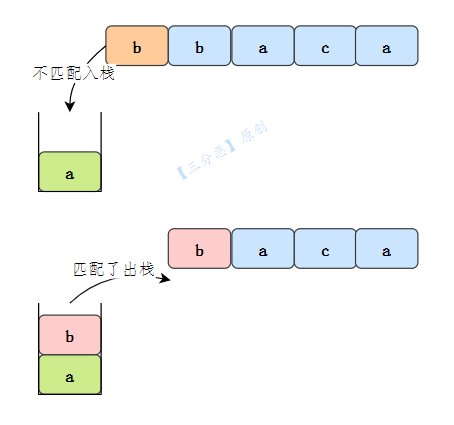``````    public String removeDuplicates(String s) {
//遍历字符串
for (int i = 0; i < s.length(); i++) {
char c = s.charAt(i);
if (stack.isEmpty() || stack.peek() != c) {
//入栈
stack.push(c);
} else {
//栈顶元素出栈
stack.pop();
}
}
//拼接栈中字符
StringBuilder str = new StringBuilder();
while (!stack.isEmpty()) {
str.append(stack.pop());
}
return str.reverse().toString();
}
``````

🚗 时间复杂度：O(n）。

🏠 空间复杂度：O(n)。

## LeetCode150. 逆波兰表达式求值

☕ 题目：150. 逆波兰表达式求值 (https://leetcode-cn.com/problems/evaluate-reverse-polish-notation/)

❓ 难度：中等

📕 描述：

• 整数除法只保留整数部分。

• 给定逆波兰表达式总是有效的。换句话说，表达式总会得出有效数值且不存在除数为 0 的情况。

``````输入：tokens = ["2","1","+","3","*"]

``````

``````输入：tokens = ["4","13","5","/","+"]

``````

``````输入：tokens = ["10","6","9","3","+","-11","*","/","*","17","+","5","+"]

((10 * (6 / ((9 + 3) * -11))) + 17) + 5
= ((10 * (6 / (12 * -11))) + 17) + 5
= ((10 * (6 / -132)) + 17) + 5
= ((10 * 0) + 17) + 5
= (0 + 17) + 5
= 17 + 5
= 22
``````

• 平常使用的算式则是一种中缀表达式，如 ( 1 + 2 ) * ( 3 + 4 ) 。
• 该算式的逆波兰表达式写法为 ( ( 1 2 + ) ( 3 4 + ) * ) 。

• 去掉括号后表达式无歧义，上式即便写成 1 2 + 3 4 + * 也可以依据次序计算出正确结果。
• 适合用栈操作运算：遇到数字则入栈；遇到算符则取出栈顶两个数字进行计算，并将结果压入栈中。

💡 思路：

`适合用栈操作运算：遇到数字则入栈；遇到算符则取出栈顶两个数字进行计算，并将结果压入栈中。`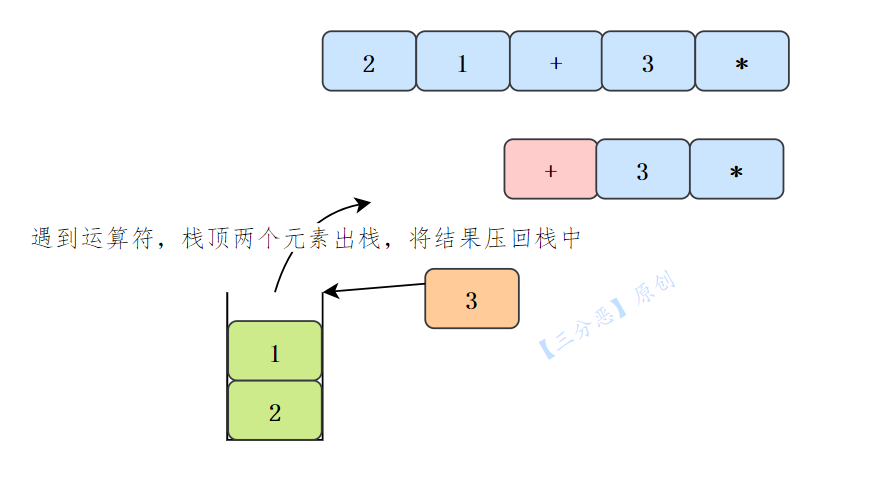``````    public int evalRPN(String[] tokens) {
for (int i = 0; i < tokens.length; i++) {
String s = tokens[i];
if (isNumber(s)) {
stack.push(Integer.parseInt(s));
} else {
int num1 = stack.pop();
int num2 = stack.pop();
if (s.equals("+")) {
stack.push(num1 + num2);
} else if (s.equals("-")) {
stack.push(num2 - num1);
} else if (s.equals("*")) {
stack.push(num1 * num2);
} else if (s.equals("/")) {
stack.push(num2 / num1);
}
}

}
return stack.pop();
}

boolean isNumber(String token) {
return !("+".equals(token) || "-".equals(token) || "*".equals(token) || "/".equals(token));
}
``````

🚗 时间复杂度：O(n）。

🏠 空间复杂度：O(n)。

## LeetCode402. 移掉 K 位数字

☕ 题目：150. 逆波兰表达式求值 (https://leetcode-cn.com/problems/evaluate-reverse-polish-notation/)

❓ 难度：中等

📕 描述：

``````输入：num = "1432219", k = 3

``````

``````输入：num = "10200", k = 1

``````

``````输入：num = "10", k = 2

``````

💡 思路：

• 遍历字符串 s，若当前栈不为空并且栈顶元素的值大于当前遍历的元素值并且移除元素的个数没有达到要求 k，则栈顶元素出栈，count 值加 1。

• 若当前遍历的元素比栈顶元素的值要大，则直接将该元素压栈。

• 若当前遍历的元素值为 " 0 " 并且栈为空，则直接跳过这次循环（要保证栈底的元素不能为 " 0 "，因为题目要求 num 不会包含任何前导零，就是不能用 " 0 " 来开头）。

• 若遍历完整个字符串而 count < k（移除的元素个数没有达到要求，示例：num = "123456", k = 3），此时直接将栈中的前三个元素依次出栈，即 " 654 " 出栈剩下的 " 321 " 翻转一下，即为最小值。

• 若当前栈为空（去掉一个最大的元素后，其余元素均为 " 0 "），则直接返回 " 0 " 即可。

``````    public String removeKdigits(String num, int k) {
if (k == num.length()) return "0";
//栈
int count = 0;
for (int i = 0; i < num.length(); i++) {
while (!stack.isEmpty() && stack.peek() > num.charAt(i) && count < k) {
stack.pop();
count++;
}
//栈为空，且当前位为0时，我们不需要将其入栈
if (num.charAt(i) == '0' && stack.isEmpty()) continue;
stack.push(num.charAt(i));
}
while (!stack.isEmpty() && count < k) {
stack.pop();
count++;
}
//这个是最后栈为空时，删除一位，比如我们的10，删除一位为0，
//按上面逻辑我们会返回""，所以我们让其返回"0"
if (stack.isEmpty()) return "0";
StringBuffer sb = new StringBuffer();
while (!stack.isEmpty()) {
sb.append(stack.pop());
}
return sb.reverse().toString();
}

``````

# 总结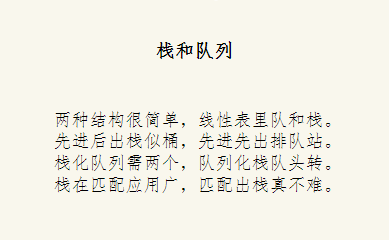`点赞``关注`不迷路，咱们下期见。